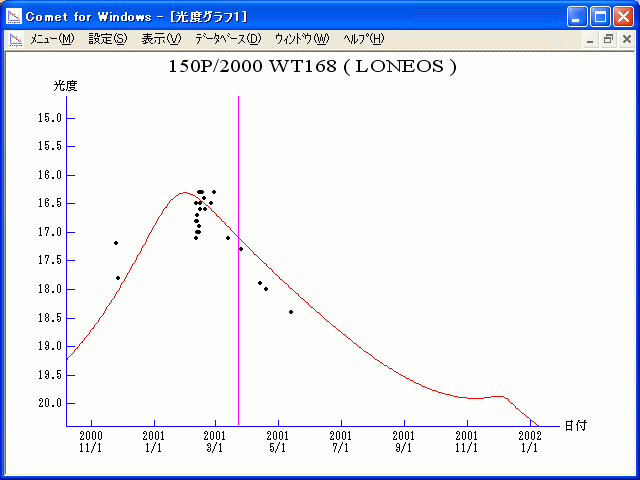# \$B%m%K%*%9WB@1(B

150P/LONEOS (2001)###\$B%W%m%U%#!<%k(B

 \$BId9f(B 150P/2000 WT168 \$BH/8+F|(B 2000\$BG/(B11\$B7n(B25,27\$BF|(B \$BH/8+8wEY(B 17.2, 17.8\$BEy(B \$BH/8+ Lowell Observatory Near-Earth Object Search, Lincoln Laboratory Near-Earth Asteroid Research project

###\$B50F;MWAG(B

```   The following improved orbital elements by Kenji Muraoka, are
from 215 observations 1978 Mar. 6 to 2001 Mar. 26, perturbations
by 9 Plantes, Moon and 5 minor planets were taken into account.
The mean residual is +/- 0.51 arc seconds.

Epoch  =  2001 Apr.  1.0  TT       JDT = 2452000.5
T  =  2001 Mar. 23.32416       +/- 0.00034 (m.e.) TT
Peri. =  245.49956                +/- 0.00013
Node  =  272.55228                +/- 0.00004   (2000.0)
Incl. =   18.51995                +/- 0.00002
q  =    1.7617180              +/- 0.0000011 AU
e  =    0.5466051              +/- 0.0000003
a  =    3.8856151              +/- 0.0000009 AU
n  =    0.12868099             +/- 0.00000004
P  =    7.659                  +/- 0.0000025  years
```

###\$B@1?^(B###\$B8wEYJQ2=(B

```        H = 14.5  G = 0.15
```##### \$B50F;MWAG\$OB<2,7r<#;a\$N7W;;\$K\$h\$k\$b\$N\$G\$9!#(B \$B@1?^\$O(B StellaNavigator Ver.2.0 for Windows (\$B%"%9%H%m%"!<%D(B \$BJTCx(B / \$B%"%9%-!<=PHG6I4)(B) \$B\$G:n@.\$7\$?\$b\$N\$G\$9!#(B \$B8wEY%0%i%U\$O(BComet for Windows\$B\$G:n@.\$7\$?\$b\$N\$G\$9!#(B